Скачать презентацию Version 1 9 2001 FINANCIAL ENGINEERING DERIVATIVES AND RISK MANAGEMENT

50606b4e2982ccb7d017d71707b24976.ppt

• Количество слайдов: 33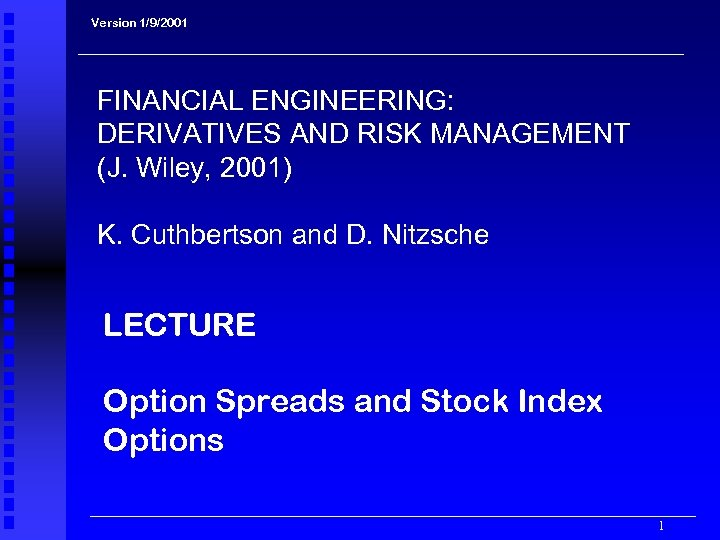Version 1/9/2001 FINANCIAL ENGINEERING: DERIVATIVES AND RISK MANAGEMENT (J. Wiley, 2001) K. Cuthbertson and D. Nitzsche LECTURE Option Spreads and Stock Index Options 1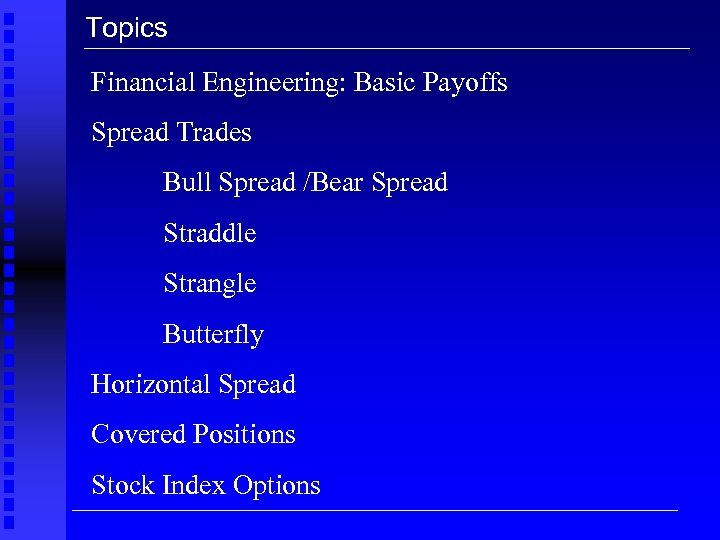Topics Financial Engineering: Basic Payoffs Spread Trades Bull Spread /Bear Spread Straddle Strangle Butterfly Horizontal Spread Covered Positions Stock Index Options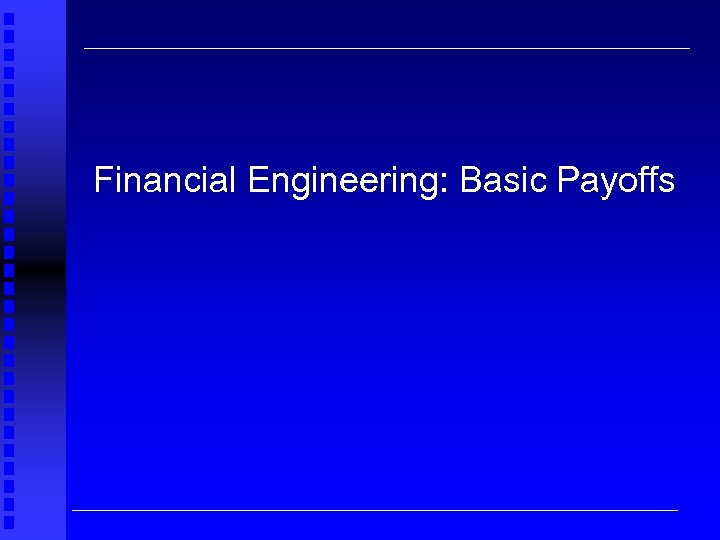Financial Engineering: Basic Payoffs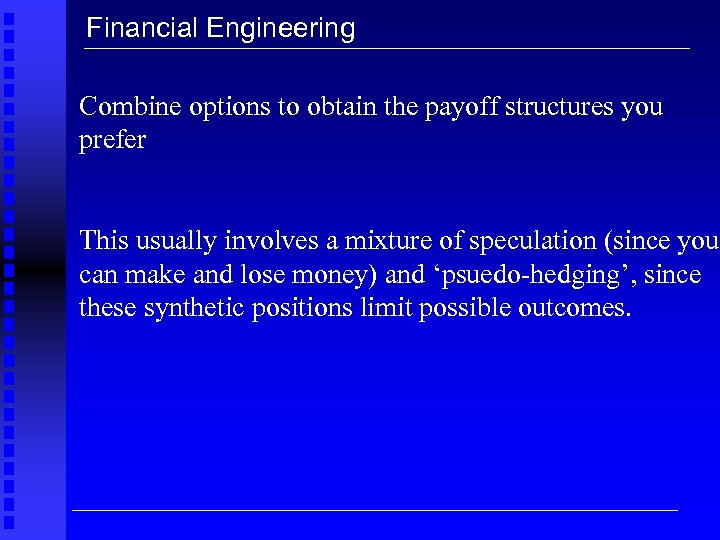Financial Engineering Combine options to obtain the payoff structures you prefer This usually involves a mixture of speculation (since you can make and lose money) and ‘psuedo-hedging’, since these synthetic positions limit possible outcomes.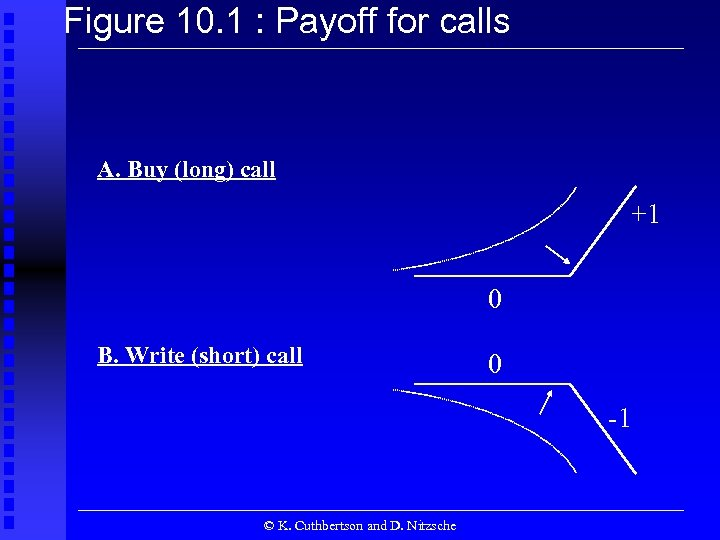Figure 10. 1 : Payoff for calls A. Buy (long) call +1 0 B. Write (short) call 0 -1 © K. Cuthbertson and D. Nitzsche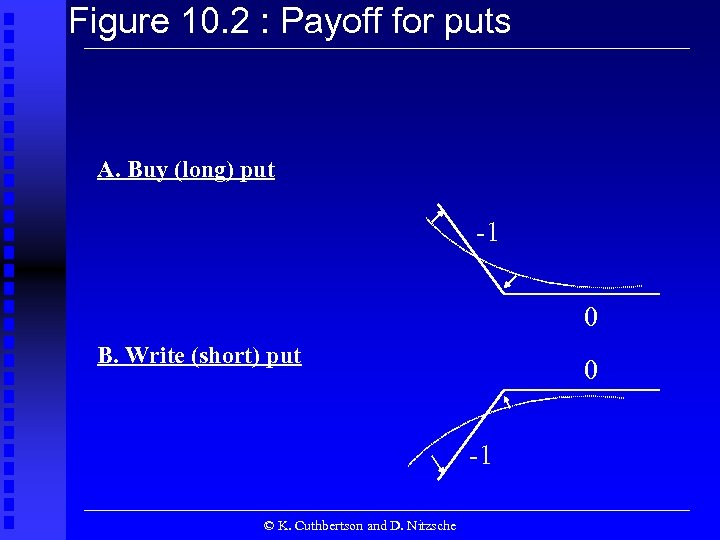Figure 10. 2 : Payoff for puts A. Buy (long) put -1 0 B. Write (short) put 0 -1 © K. Cuthbertson and D. Nitzsche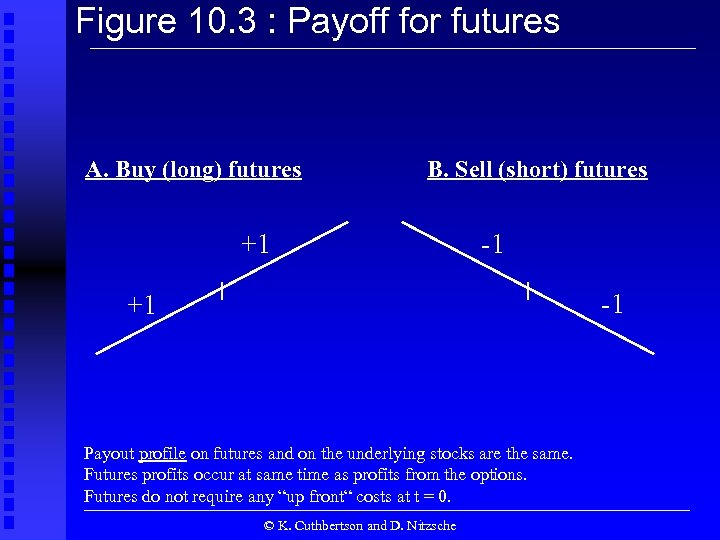Figure 10. 3 : Payoff for futures A. Buy (long) futures B. Sell (short) futures +1 -1 -1 +1 Payout profile on futures and on the underlying stocks are the same. Futures profits occur at same time as profits from the options. Futures do not require any “up front“ costs at t = 0. © K. Cuthbertson and D. Nitzsche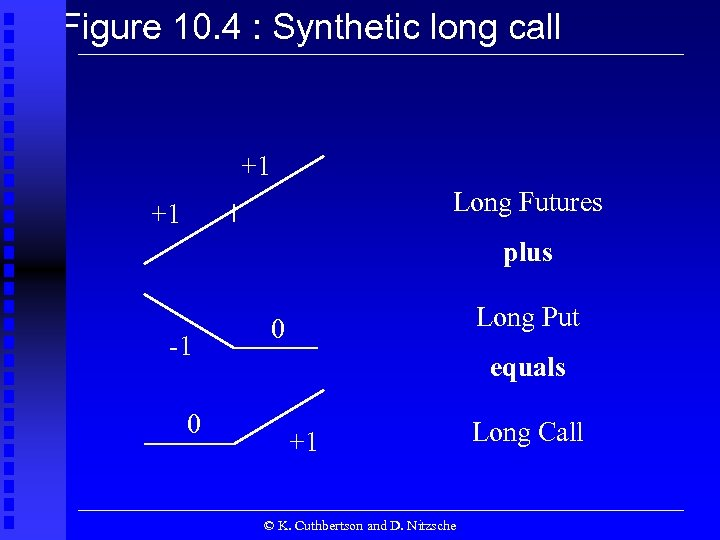Figure 10. 4 : Synthetic long call +1 Long Futures +1 plus -1 0 Long Put 0 equals +1 © K. Cuthbertson and D. Nitzsche Long Call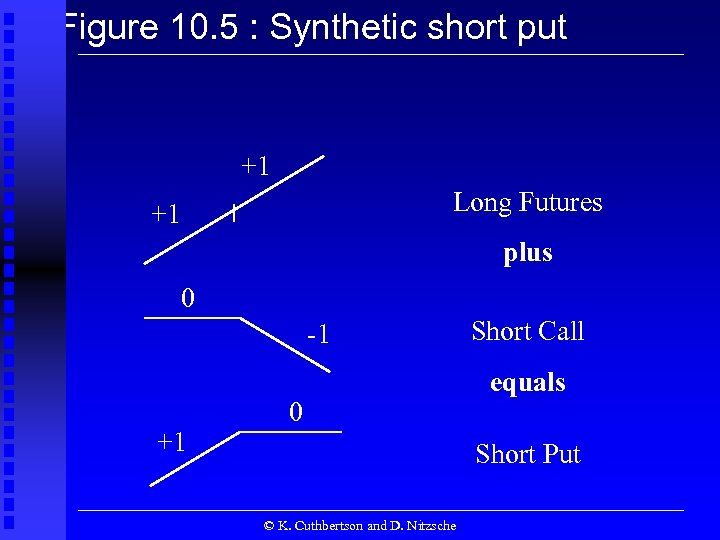Figure 10. 5 : Synthetic short put +1 Long Futures +1 plus 0 -1 +1 0 Short Call equals Short Put © K. Cuthbertson and D. Nitzsche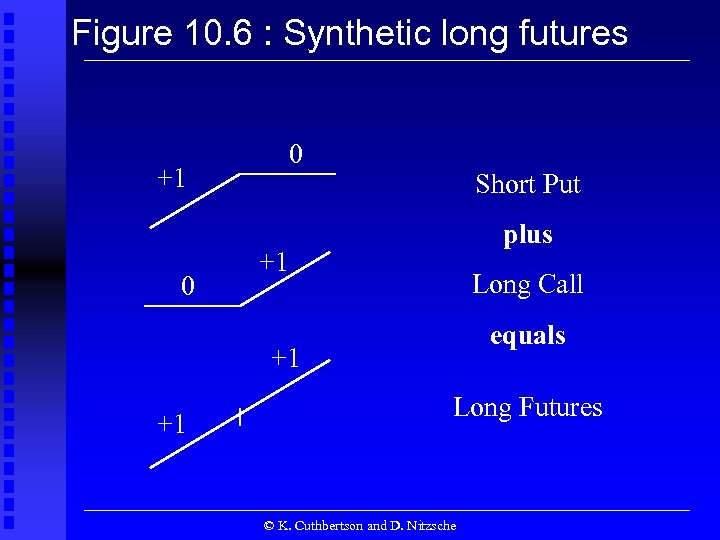Figure 10. 6 : Synthetic long futures 0 +1 0 Short Put plus +1 Long Call equals +1 +1 Long Futures © K. Cuthbertson and D. Nitzsche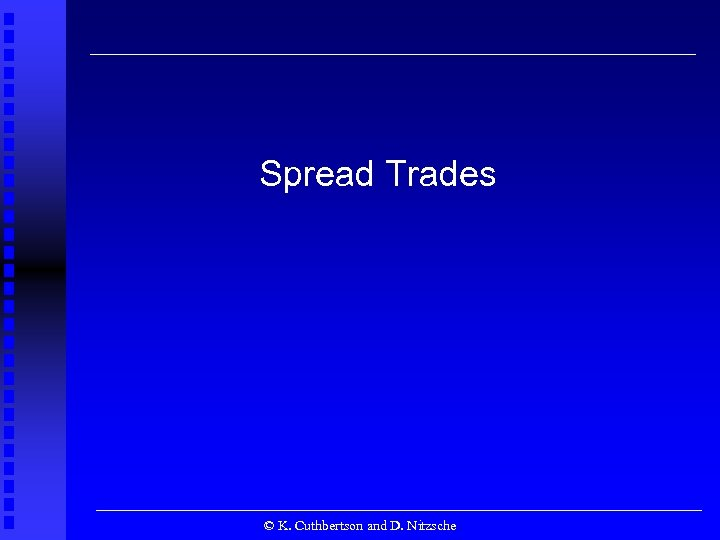Spread Trades © K. Cuthbertson and D. Nitzsche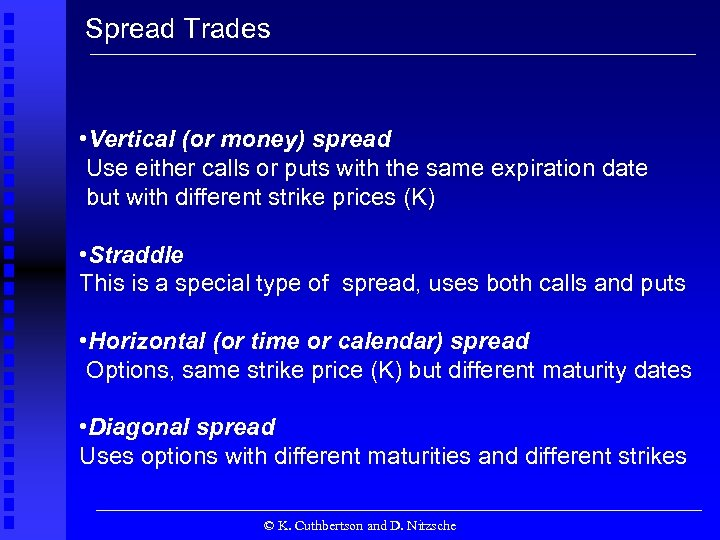Spread Trades • Vertical (or money) spread Use either calls or puts with the same expiration date but with different strike prices (K) • Straddle This is a special type of spread, uses both calls and puts • Horizontal (or time or calendar) spread Options, same strike price (K) but different maturity dates • Diagonal spread Uses options with different maturities and different strikes © K. Cuthbertson and D. Nitzsche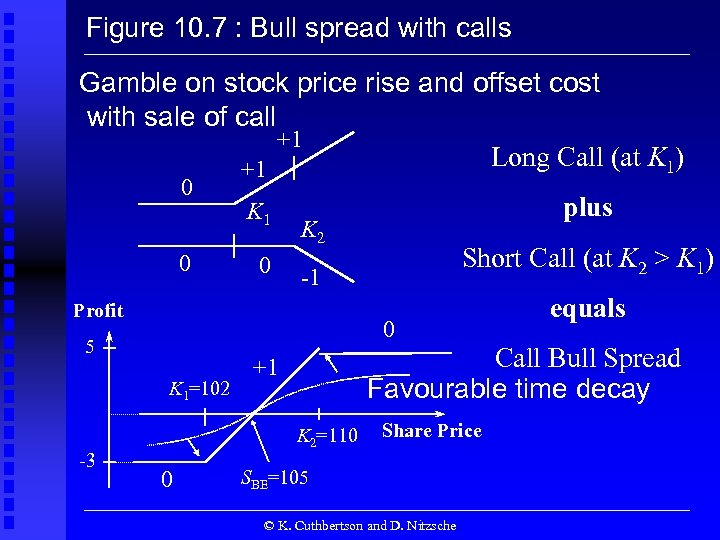Figure 10. 7 : Bull spread with calls Gamble on stock price rise and offset cost with sale of call +1 Long Call (at K 1) +1 0 K 2 Short Call (at K 2 > K 1) -1 0 Profit 0 5 K 1=102 equals Call Bull Spread Favourable time decay +1 K 2=110 0 -3 plus Share Price SBE=105 © K. Cuthbertson and D. Nitzsche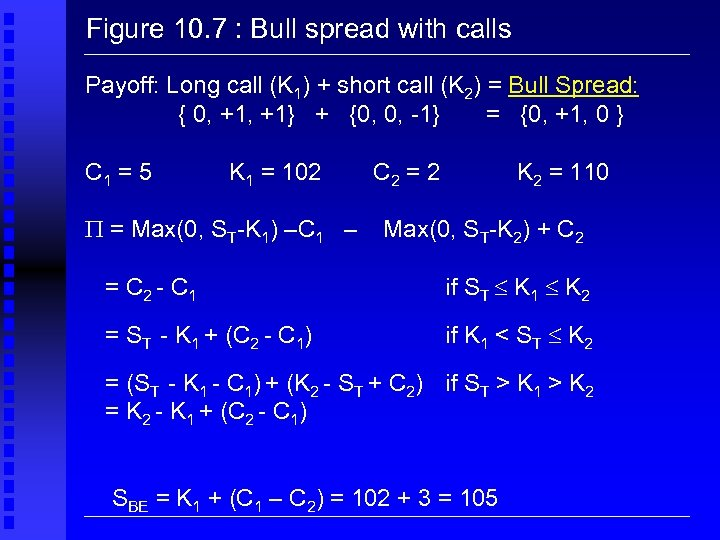Figure 10. 7 : Bull spread with calls Payoff: Long call (K 1) + short call (K 2) = Bull Spread: { 0, +1} + {0, 0, -1} = {0, +1, 0 } C 1 = 5 K 1 = 102 = Max(0, ST-K 1) –C 1 – C 2 = 2 K 2 = 110 Max(0, ST-K 2) + C 2 = C 2 - C 1 if ST K 1 K 2 = ST - K 1 + (C 2 - C 1) if K 1 < ST K 2 = (ST - K 1 - C 1) + (K 2 - ST + C 2) if ST > K 1 > K 2 = K 2 - K 1 + (C 2 - C 1) SBE = K 1 + (C 1 – C 2) = 102 + 3 = 105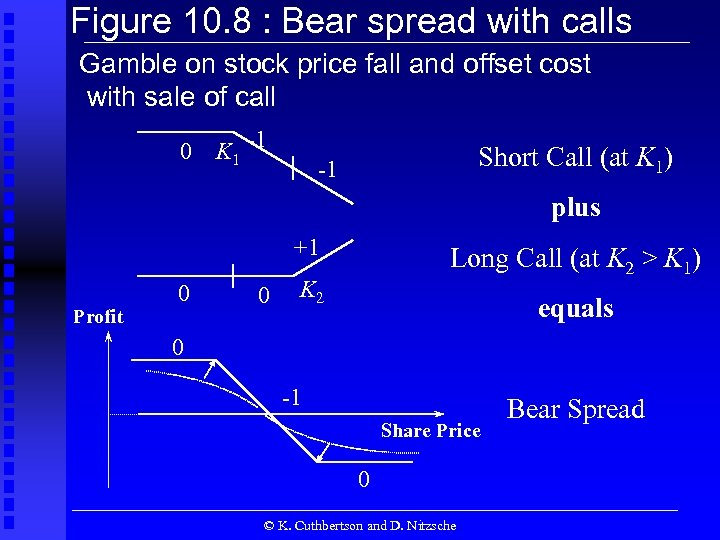Figure 10. 8 : Bear spread with calls Gamble on stock price fall and offset cost with sale of call 0 K 1 -1 Short Call (at K 1) -1 plus +1 0 K 2 equals 0 -1 Share Price 0 Profit 0 Long Call (at K 2 > K 1) © K. Cuthbertson and D. Nitzsche Bear Spread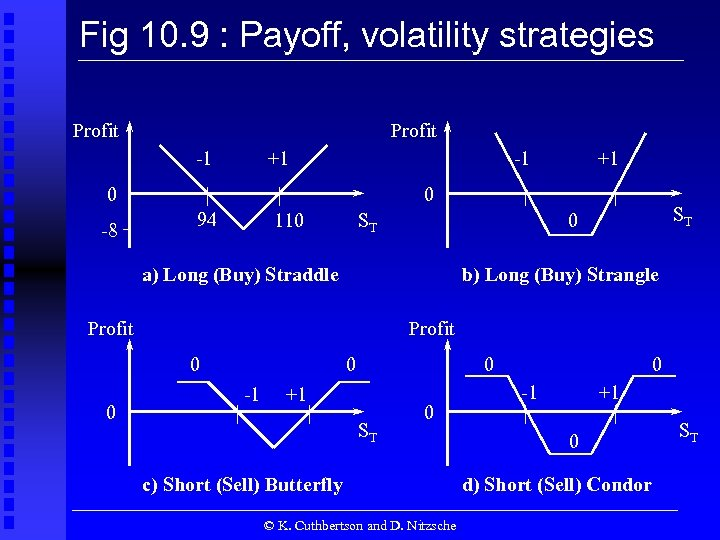Fig 10. 9 : Payoff, volatility strategies Profit -1 +1 -1 0 -8 0 94 110 ST ST 0 a) Long (Buy) Straddle b) Long (Buy) Strangle Profit 0 0 +1 0 -1 0 +1 ST 0 c) Short (Sell) Butterfly © K. Cuthbertson and D. Nitzsche 0 -1 +1 0 d) Short (Sell) Condor ST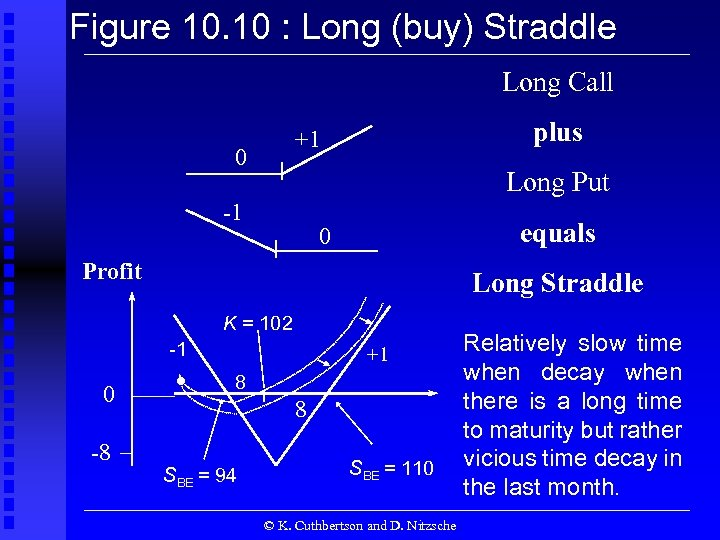Figure 10. 10 : Long (buy) Straddle Long Call plus +1 0 Long Put -1 equals 0 Profit Long Straddle K = 102 -1 0 -8 . +1 8 8 SBE = 94 SBE = 110 © K. Cuthbertson and D. Nitzsche Relatively slow time when decay when there is a long time to maturity but rather vicious time decay in the last month.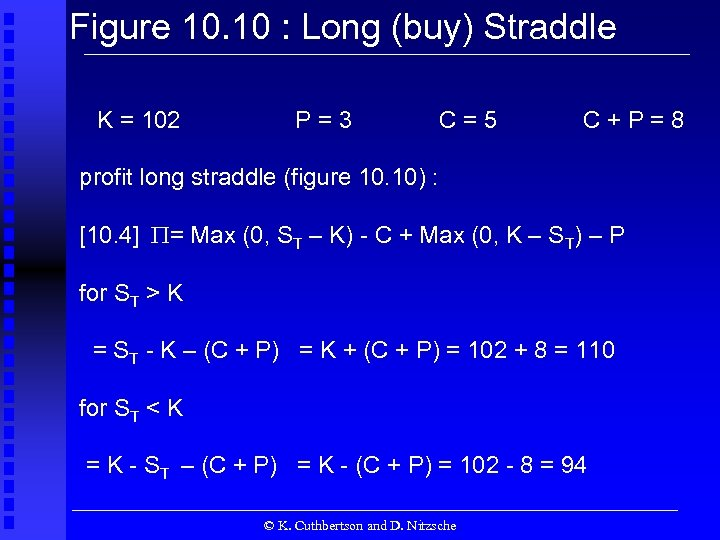Figure 10. 10 : Long (buy) Straddle K = 102 P=3 C=5 C+P=8 profit long straddle (figure 10. 10) : [10. 4] = Max (0, ST – K) - C + Max (0, K – ST) – P for ST > K = ST - K – (C + P) = K + (C + P) = 102 + 8 = 110 for ST < K = K - ST – (C + P) = K - (C + P) = 102 - 8 = 94 © K. Cuthbertson and D. Nitzsche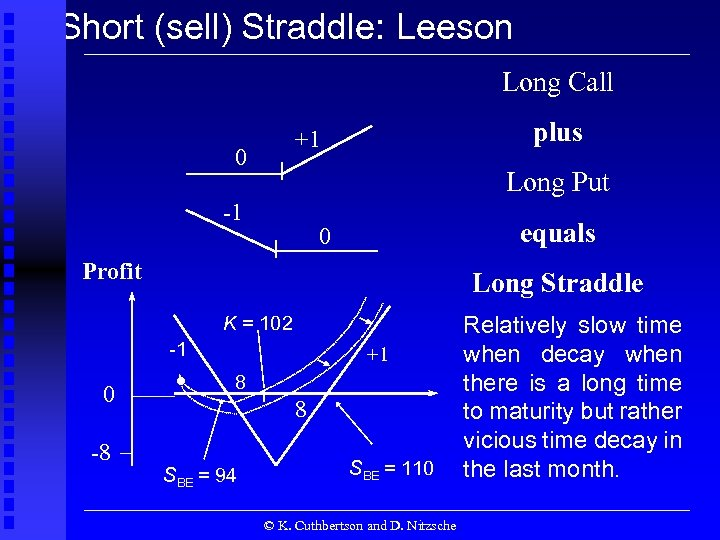Short (sell) Straddle: Leeson Long Call plus +1 0 Long Put -1 equals 0 Profit Long Straddle K = 102 -1 0 -8 . +1 8 8 SBE = 94 SBE = 110 © K. Cuthbertson and D. Nitzsche Relatively slow time when decay when there is a long time to maturity but rather vicious time decay in the last month.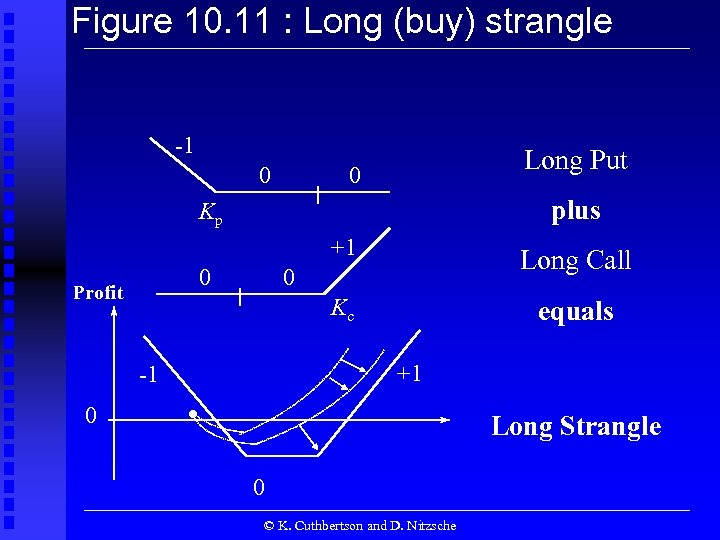Figure 10. 11 : Long (buy) strangle -1 0 Long Put 0 plus Kp +1 Kc 0 Profit -1 0 Long Call equals 0 +1 . Long Strangle 0 © K. Cuthbertson and D. Nitzsche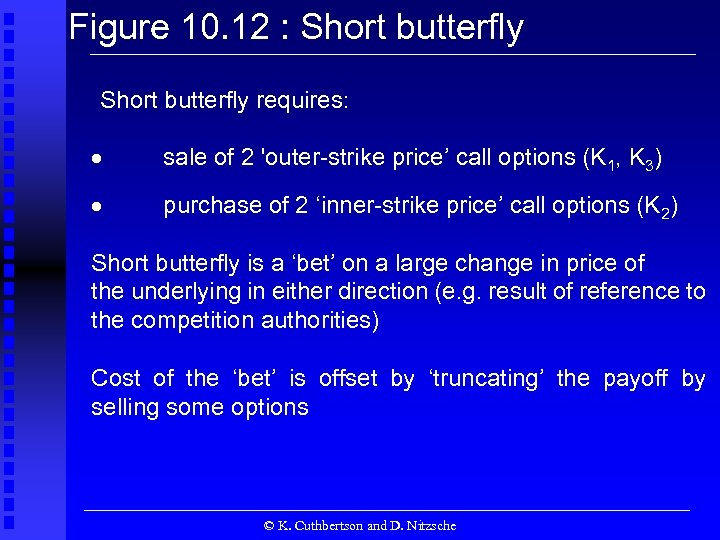Figure 10. 12 : Short butterfly requires: sale of 2 'outer-strike price’ call options (K 1, K 3) purchase of 2 ‘inner-strike price’ call options (K 2) Short butterfly is a ‘bet’ on a large change in price of the underlying in either direction (e. g. result of reference to the competition authorities) Cost of the ‘bet’ is offset by ‘truncating’ the payoff by selling some options © K. Cuthbertson and D. Nitzsche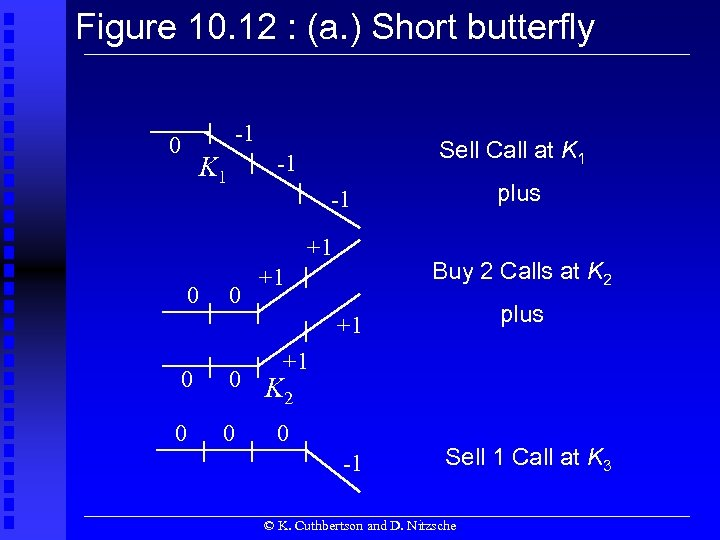Figure 10. 12 : (a. ) Short butterfly -1 0 Sell Call at K 1 -1 K 1 +1 0 plus -1 0 Buy 2 Calls at K 2 +1 +1 plus -1 Sell 1 Call at K 3 +1 0 0 K 2 0 0 0 © K. Cuthbertson and D. Nitzsche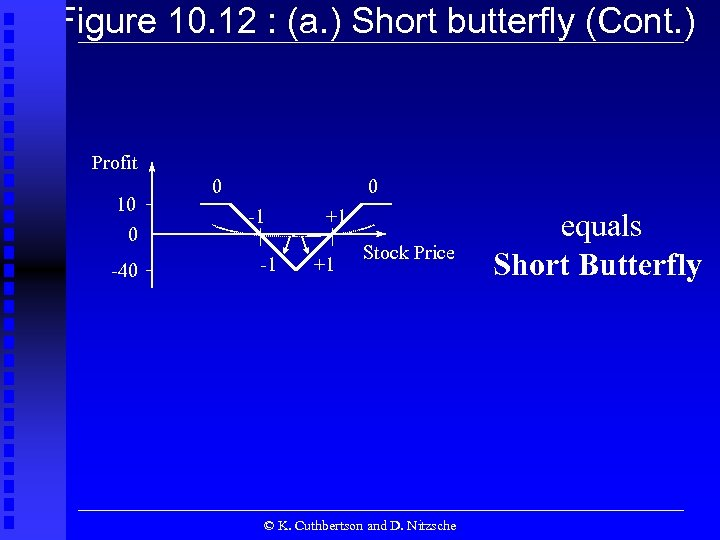Figure 10. 12 : (a. ) Short butterfly (Cont. ) Profit 10 0 -40 0 0 -1 -1 +1 +1 Stock Price © K. Cuthbertson and D. Nitzsche equals Short Butterfly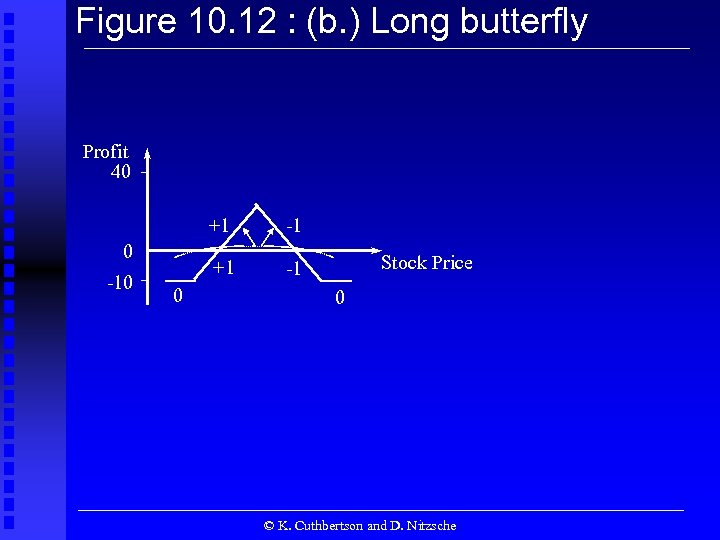Figure 10. 12 : (b. ) Long butterfly Profit 40 +1 +1 0 -10 0 -1 -1 Stock Price 0 © K. Cuthbertson and D. Nitzsche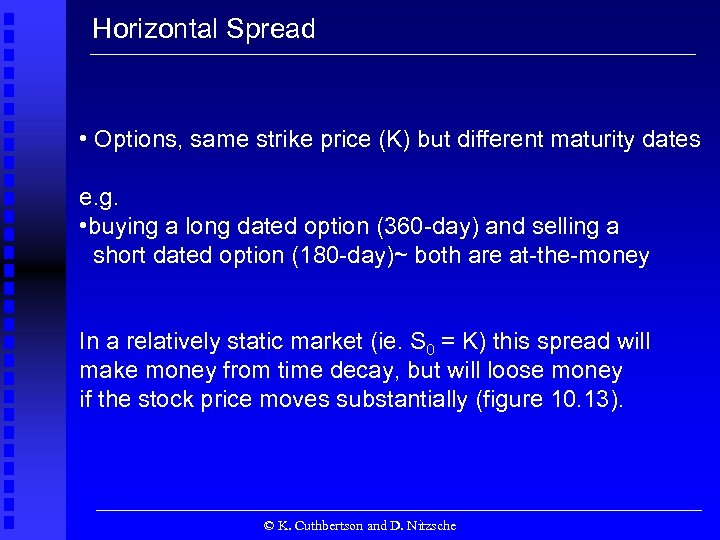Horizontal Spread • Options, same strike price (K) but different maturity dates e. g. • buying a long dated option (360 -day) and selling a short dated option (180 -day)~ both are at-the-money In a relatively static market (ie. S 0 = K) this spread will make money from time decay, but will loose money if the stock price moves substantially (figure 10. 13). © K. Cuthbertson and D. Nitzsche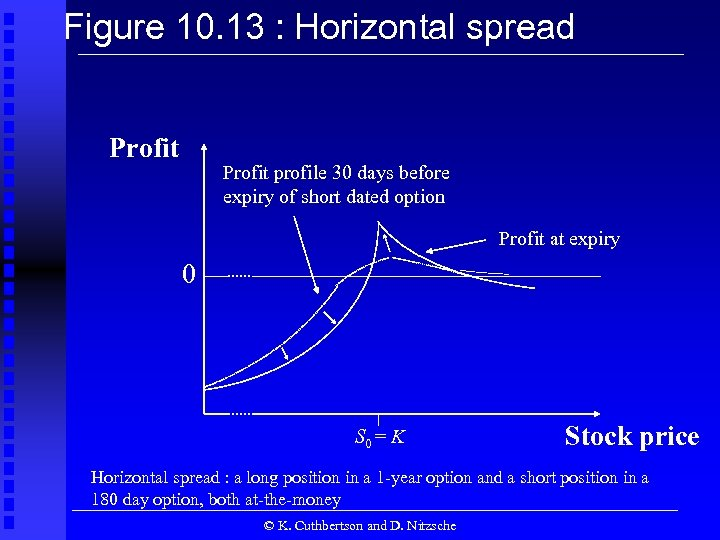Figure 10. 13 : Horizontal spread Profit profile 30 days before expiry of short dated option Profit at expiry 0 S 0 = K Stock price Horizontal spread : a long position in a 1 -year option and a short position in a 180 day option, both at-the-money © K. Cuthbertson and D. Nitzsche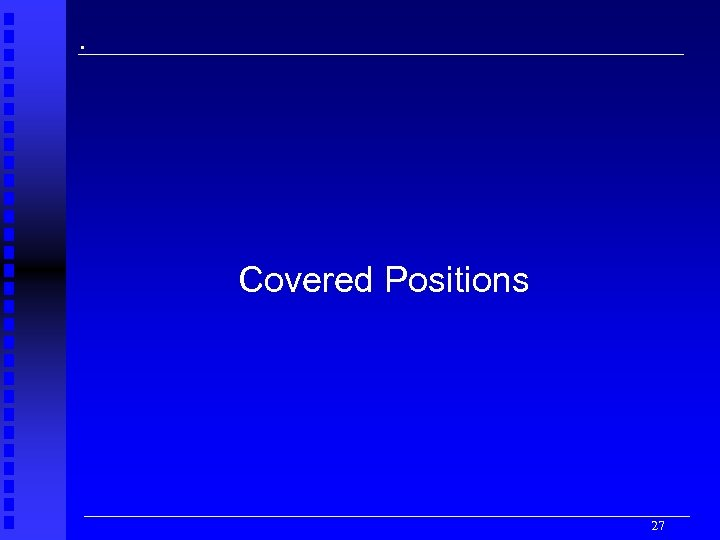. Covered Positions 27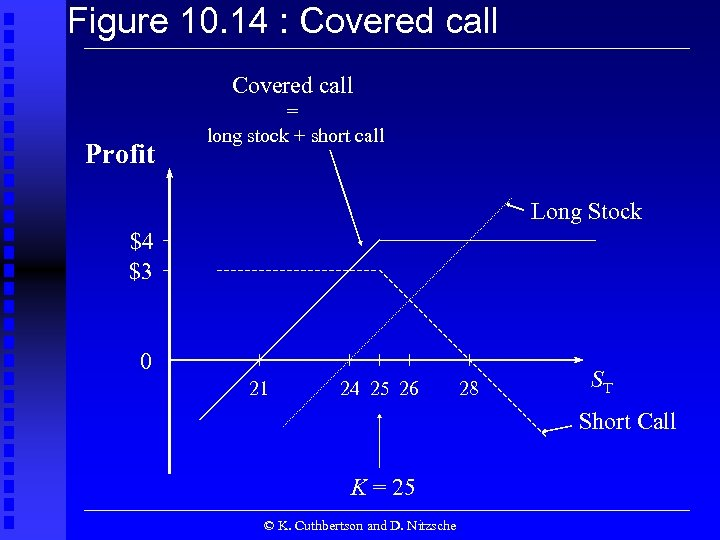Figure 10. 14 : Covered call Profit = long stock + short call Long Stock \$4 \$3 0 21 24 25 26 28 ST Short Call K = 25 © K. Cuthbertson and D. Nitzsche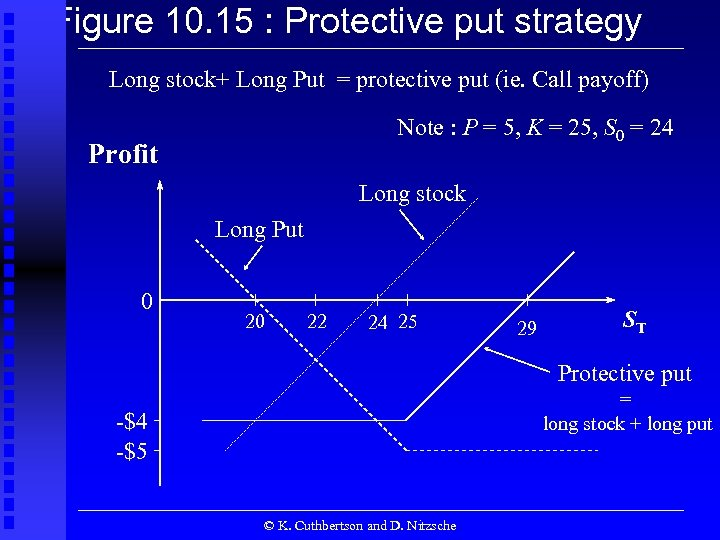Figure 10. 15 : Protective put strategy Long stock+ Long Put = protective put (ie. Call payoff) Note : P = 5, K = 25, S 0 = 24 Profit Long stock Long Put 0 20 22 24 25 29 ST Protective put = long stock + long put -\$4 -\$5 © K. Cuthbertson and D. Nitzsche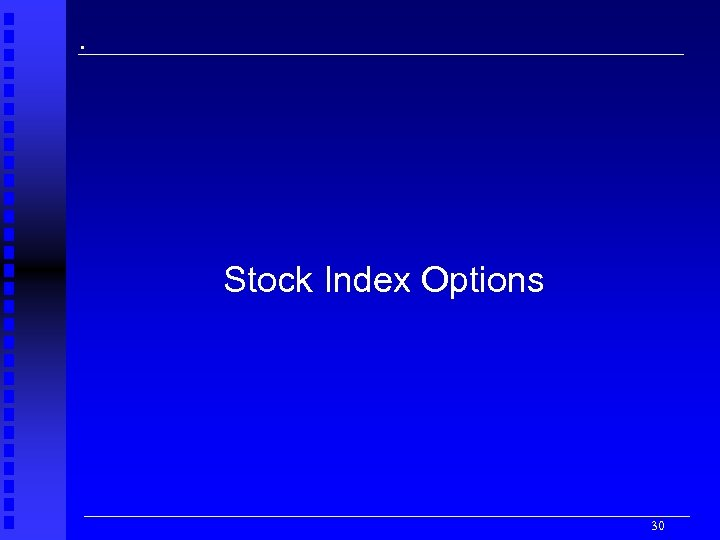. Stock Index Options 30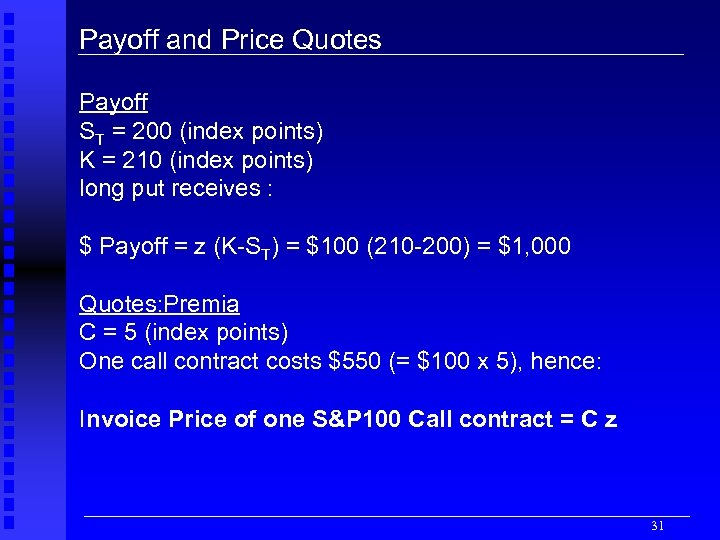Payoff and Price Quotes Payoff ST = 200 (index points) K = 210 (index points) long put receives : \$ Payoff = z (K-ST) = \$100 (210 -200) = \$1, 000 Quotes: Premia C = 5 (index points) One call contract costs \$550 (= \$100 x 5), hence: Invoice Price of one S&P 100 Call contract = C z 31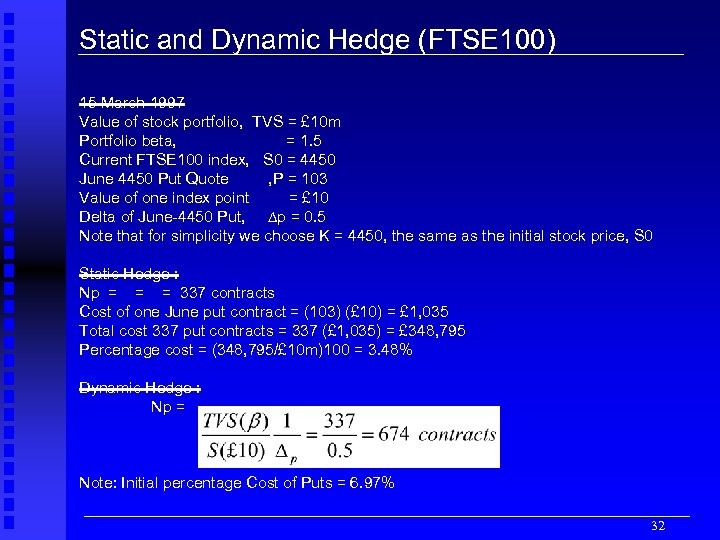Static and Dynamic Hedge (FTSE 100) 15 March 1997 Value of stock portfolio, TVS = £ 10 m Portfolio beta, = 1. 5 Current FTSE 100 index, S 0 = 4450 June 4450 Put Quote , P = 103 Value of one index point = £ 10 Delta of June-4450 Put, p = 0. 5 Note that for simplicity we choose K = 4450, the same as the initial stock price, S 0 Static Hedge : Np = = = 337 contracts Cost of one June put contract = (103) (£ 10) = £ 1, 035 Total cost 337 put contracts = 337 (£ 1, 035) = £ 348, 795 Percentage cost = (348, 795/£ 10 m)100 = 3. 48% Dynamic Hedge : Np = Note: Initial percentage Cost of Puts = 6. 97% 32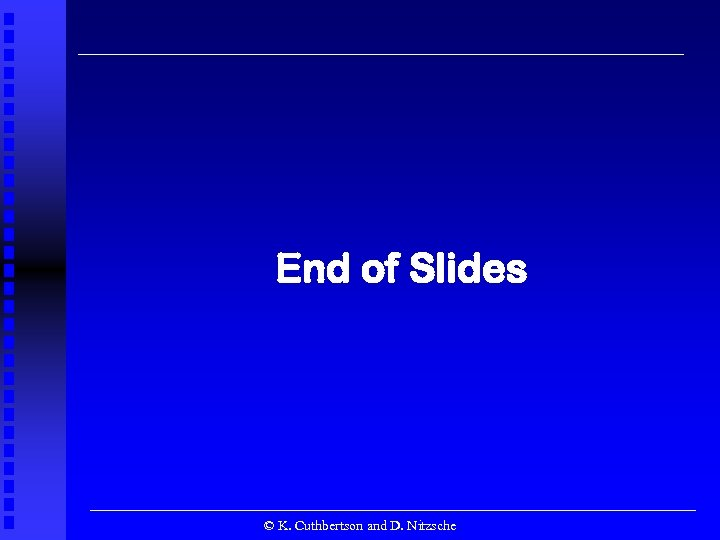End of Slides © K. Cuthbertson and D. Nitzsche